What Is ROC Curve in Machine Learning using Python? ROC Curve Example*Subject to Terms and ConditionUpdated on 28th Jun, 23 1.8 K Views

## Introduction to ROC Curve in Machine Learning

Let’s start our ROC Curve in machine learning blog with the ROC curve full form, which is Receiver Operating Characteristic curve. It is nothing but a graph displaying the performance of a classification model. It is a very popular method to measure the accuracy of a classification model. These are the topics covered in this blog.

Here’s a video from Intellipaat on ROC curve in Machine Learning with R.

These are the topics covered in this ROC Curve in Machine Learning blog:

## Thresholding in Machine Learning Classifier Model

We know that logistic regression gives us the result in the form of probability. Say, we are building a logistic regression model to detect whether breast cancer is malignant or benign. A model that returns probability of 0.8 for a particular patient, that means the patient is more likely to have malignant breast cancer. On the other hand, another patient with a prediction score of 0.2 on that same logistic regression model is very likely not to have malignant breast cancer.

Then, what about a patient with a prediction score of 0.6? In this scenario, we must define a classification threshold to map the logistic regression values into binary categories. For instance, all values above that threshold would indicate ‘malignant’ and values below that threshold would indicate ‘benign.’

By default, the logistic regression model assumes the classification threshold to be 0.5, but thresholds are completely problem dependent. In order to achieve the desired output, we can tune the threshold.

Let’s say, sensitivity of a metal detector depends on the threshold value in order to detect metals.

If we need to detect big metals, then we need to increase the threshold so that the sensitivity gets decreased and the metal detector doesn’t go off near small metals.

But if we need to detect small metals, then we need to lower the threshold, so that the sensitivity increases and buzzer can go off near small metals as well.

But now the question is how do we tune the threshold? How do we know which threshold would give us more accurate logistic regression model? So, for that we will be using the ROC curve and the Area Under ROC Curve (AUC). Let us go ahead and understand what ROC curve is and how do we use that in machine learning.

For the best of career growth, check out Intellipaat’s Machine Learning Course and get certified.

## What is ROC Curve?

ROC or Receiver Operating Characteristic plot is used to visualise the performance of a binary classifier. It gives us the trade-off between the True Positive Rate (TPR) and the False Positive Rate (FPR) at different classification thresholds.

### True Positive Rate:

True Positive Rate is the proportion of observations that are correctly predicted to be positive.

### False Positive Rate:

False Positive Rate is the proportion of observations that are incorrectly predicted to be positive.

For different threshold values we will get different TPR and FPR. So, in order to visualise which threshold is best suited for the classifier we plot the ROC curve. The following figure shows what a typical ROC curve look like.

Alright, now that we know the basics of ROC curve let us see how it helps us measuring performance of a classifier.

Get 100% Hike!

Master Most in Demand Skills Now !

## ROC Curve of a Random Classifier Vs. a Perfect Classifier

The ROC curve of a random classifier with the random performance level (as shown below) always shows a straight line. This random classifier ROC curve is considered to be the baseline for measuring the performance of a classifier. Two areas separated by this ROC curve indicates an estimation of the performance level—good or poor.

ROC curves that fall under the area at the top-left corner indicate good performance levels, whereas ROC curves fall in the other area at the bottom-right corner indicate poor performance levels. An ROC curve of a perfect classifier is a combination of two straight lines both moving away from the baseline towards the top-left corner.

Now, we might be wondering how a perfect classifier looks like.

Note: The dotted line represents the ROC curve of a purely random classifier; a good classifier stays as far away from that line as possible (toward the top-left corner).

## Area Under ROC Curve

Area Under the Curve or AUC ROC curve is nothing but the area under the curve calculated in the ROC space. One of the easy ways to calculate the AUC score is using the trapezoidal rule, which is adding up all trapezoids under the curve.

Although the theoretical range of the AUC ROC curve score is between 0 and 1, the actual scores of meaningful classifiers are greater than 0.5, which is the AUC ROC curve score of a random classifier.

## ROC Curve in Machine Learning with Python

In order to execute ROC in Machine Learning we will be using Python programming. Also, we will be taking reference from the confusion matrix example. Refer to Confusion Matrix blog for prior steps.

Recap: In the Confusion Matrix example, we built a logistic regression classifier to predict whether the state of breast cancer is malignant or benign. We observed the confusion matrix in python as shown below.

`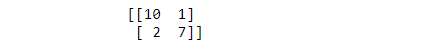`
• Let us see the interpretation of the classifier we built over there in ROC curve.
• Step 1: Import the roc python libraries and use roc_curve() to get the threshold, TPR, and FPR.
`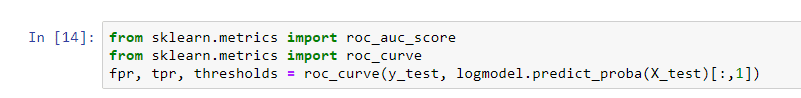`
• Take a look at the FPR, TPR, and threshold array:
`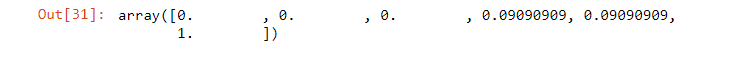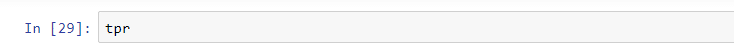`
`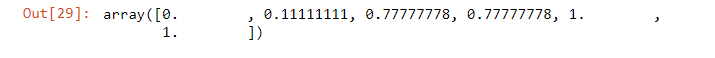`
`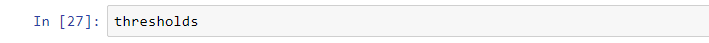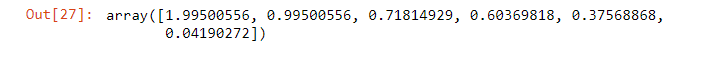`

• Step 2: For AUC use roc_auc_score() python function for ROC
•• Step 3: Plot the ROC curve
`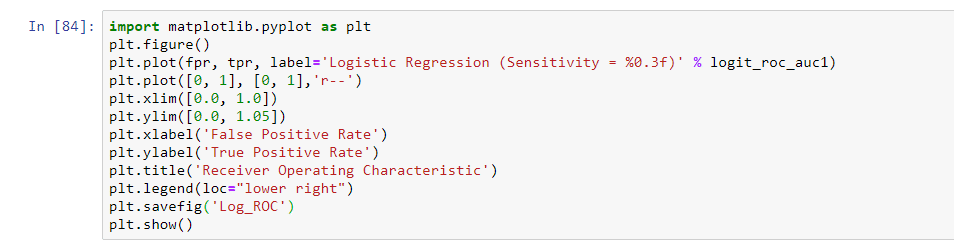`
• `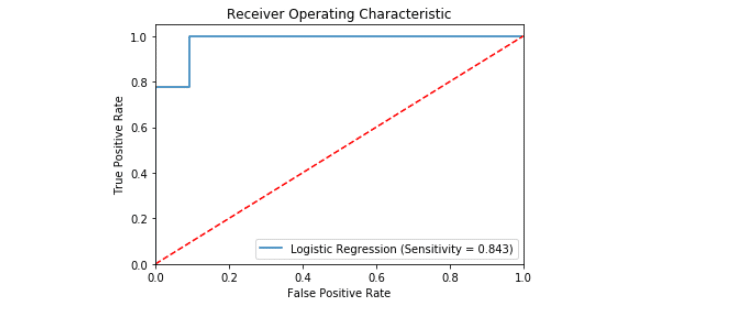`
• Now we will be tuning the threshold value to build a classifier model with more desired output.
• Step 4:  Print the predicted probabilities of class 1 (malignant cancer)
`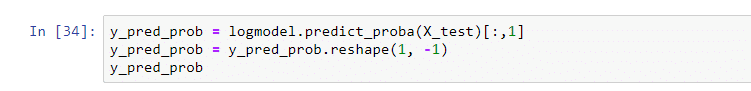`
`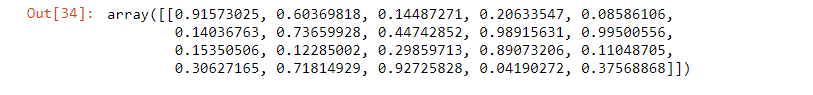`
• Step 5: Set the threshold at 0.35
`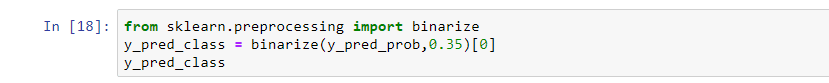`
• ``
`Converting the array from float data type to integer data type.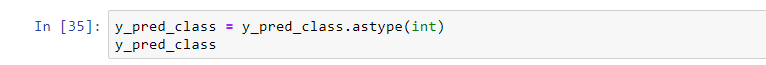`

Become Master of Machine Learning by going through this online Machine Learning course in Pune.

• Step 6: Print out the new Confusion Matrix
`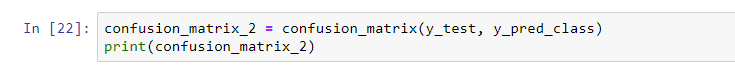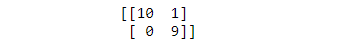`
• Note:Here,
• True Positive is 10
• True Negative is 9
• False Positive is 1
• False Negative is 0

As you can see, clearly the classifier has improved. Compare it with the previous Confusion Matrix given below:

``
• Step 7: Print out other performance metrics
`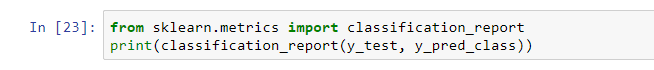`
• `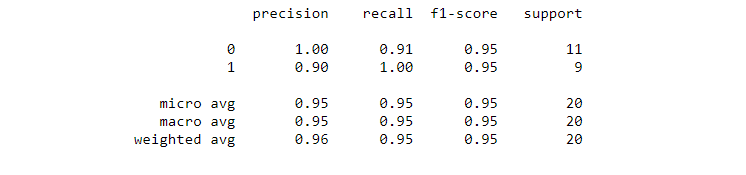`
•
• Compare the performance metrics (above) at threshold 0.3 to the performance metrics at default threshold (below).
•
• `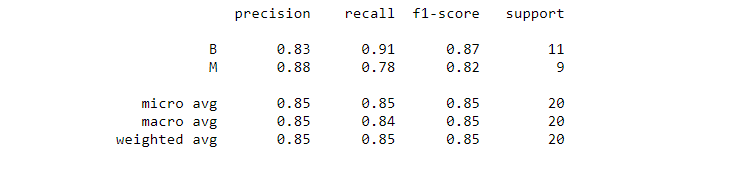`
• In order to see how sensitivity changes with threshold, let us plot the ROC curve again.
`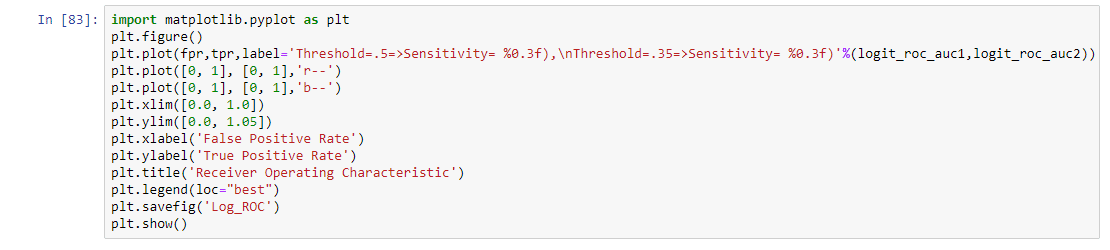`
`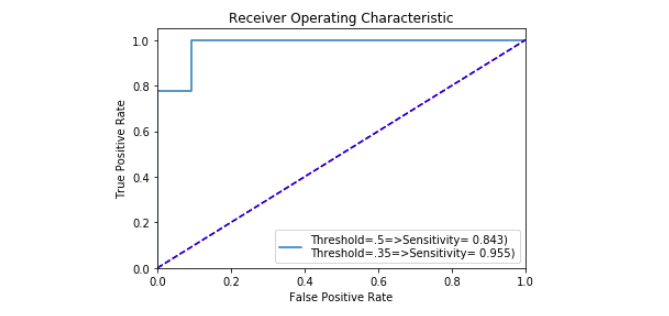`

## What Did We Learn So Far?

In this blog, we have discussed what thresholding is and how thresholding tuning helps better the classifier according our need. We have also discussed use of ROC curve in machine learning and how it works with an ROC curve example. We also talked about the area under the curve or AUC. Hope you found this blog helpful. See you in the next one.

If you have any queries related to Machine learning, you can post them on our Machine Learning Community, and our team of experts will resolve them for you!

Course Schedule

Name Date Details
Machine Learning Course 30 Sep 2023(Sat-Sun) Weekend Batch
View Details
Machine Learning Course 07 Oct 2023(Sat-Sun) Weekend Batch
View Details
Machine Learning Course 14 Oct 2023(Sat-Sun) Weekend Batch
View Details